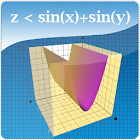trinomial factoring graphing 3d calculator

All Android applications categories

All Android games categories# trinomial factoring graphing 3d calculator

0 0

## Screenshots

Description

Algebra Calculator that will help you solve any equation with work solutions and steps to solve all types of equations also works offline !
Computer science: provides basic and advanced mathematical functions useful for school or college. also with a graphing calculator + math algebra & calculus
with a unit converter works also for civil engineering
Simplify expressions easily with our smart calculator like radical expressions
You can access the polynomial long division calculator. This cheat calculator can help you with your math homework. with it remembering and showing history for your past equations